Courses

# Random Processes & Variables GATE Notes | EduRev

## GATE : Random Processes & Variables GATE Notes | EduRev

The document Random Processes & Variables GATE Notes | EduRev is a part of the GATE Course Communication System.
All you need of GATE at this link: GATE

In this article, you will find the Study Notes on Random Processes & Variables which will cover the topics such as Introduction to Random Process and variables, Mean Function, Auto-correlation function, Properties of Auto Correction Function, Stationary Process, Power and Energy of Random Signal, Cross-Correlation, Random Processes and Linear Systems, Power Spectral Density.

Introduction to Random Process and variables:

• The concept of a random process allows us to study systems involving signals that are not entirely predictable.
• A random process is the natural extension of the concept of a random variable when dealing with signals.
• A random process is a collection of time functions or signals corresponding to various outcomes of a random experiment.
• Random process represents the mathematical model of these random signals.
• A random process (or stochastic process) is a collection of random variables (functions) indexed by time.
• Random process can be denoted by X(t,s) or X(t), where s is the sample point of the random experiment and t is the time.
• A random variable is an outcome is mapped to a number whereas the Random process is an outcome is mapped to a random waveform that is a function of time.
• These random signals play a fundamental role in the fields of communications, signal processing, control systems, and many other engineering disciplines.

Types of classes of signals in deterministic signals:

1. Continuous time and continuous amplitude signals:These are a function of a continuous independent variable, time. The range of the amplitude of the function is also continuous.
2. Continuous time and discrete amplitude signals: These are a function of a continuous independent variable, time—but the amplitude is discrete.
3. Discrete time and continuous amplitude signals: These are functions of a quantized or discrete independent time variable, while the range of amplitudes is continuous.
4. Discrete time and discrete amplitude signals: These are functions where both the independent time variable and the amplitude are discrete.

Mean Function

The mean function of a random process is the expected value of the process.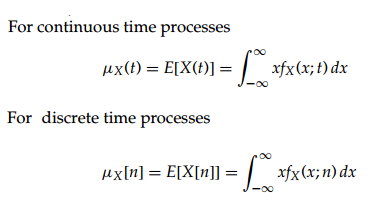Auto-correlation function:

• This represents the relation of a function with its shifted version.
• The autocorrelation function of the random process X(t), denoted by Rxx(t1 ,t2).
• Auto correlation function is defined as the expected value of the product of X(t1) ,X(t2)
• Rxx(t1, t2) = E [X(t1) X(t2)]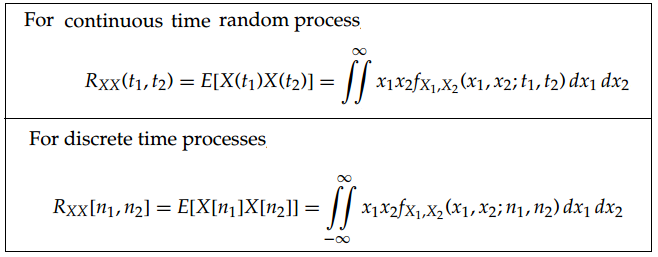Properties of Auto Correction Function:

Rx (τ) is the auto correction function of stationary process X(t) has following properties.

• Rx (τ) is an even function i.e., Rx (τ) = Rx (–τ)
• The maximum absolute value of Rx (τ) is achieved at τ = 0 i.e., | Rx (τ)| ≤ Rx (0)
• If for some T0 we have Rx(T0) = Rx(0), then for all integers K, Rx(KT0) = Rx (0).

Stationary Process:

A process whose statistical properties are independent of a choice of time origin is called as a stationary process.

• A random process is considered stationary (in the strict sense) if its specification is independent of time, i.e., if the joint probability density function of its sample values is independent of time.
• A process X(t) is called as strict-sense stationary if the statistics of the process are invariant to a time shift.
• A process X(t) is called as wide-sense stationary (WSS) if the mean function and autocorrelation function are invariant to a time shift.
• mx (t) = E [X(t)] = constant (independent of t)
• Rxx (t1t2) depends only on the time difference τ = (t1 – t2) and not on t1 and t2 individually. Rxx (t + τ, t) = Rxx (τ)
• All strict sense stationary random processes are also WSS, provided that the mean and autocorrelation function exist.
• If mx(t) and Rxx (t + τ, t) are periodic with period t, the process is called as the cyclostationary process.
• Rx(0) gives power content of a power signal (if signal is power signal).
• Rx(0) gives energy content of the signal (if a signal is energy signal).
• Fourier transform of Rxx(τ) gives power spectral density for power signal and energy spectral density for energy signal.

Power and Energy of Random Signal:

Power content of a random signal X is given by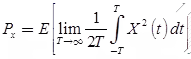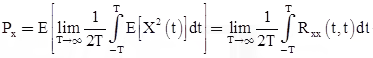Energy content of random signal is given by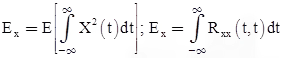For the case of stationary processes, only power type process is of theoretical and practical interest.

Cross-Correlation:

The cross correlation function between two random processes X(t) and Y(t) is

Rx,y(t1, t2) = E[X (t1) Y (t2)];

Rx,y(t1, t2) = Ry,x (t2, t1)

Random Processes and Linear Systems:

Let random process X(t) is input to an LTI system having impulse response h(t) and y(t) is output of LTI system then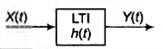• If X(t) is stationary process then X(t) and Y(t) will be jointly stationary with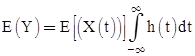Rxy(τ) = Rx(τ) * h(–τ)

Rx(τ) * h(–τ)

Ry(τ) = Rx(τ) * h(–τ) * h(τ)

Sy(f) = Sx(f)|H(f)|2

where * represents convolution

Power Spectral Density:

• A quantity that is related to the auto-correlation function is the power spectral density [PSD, Sxx(f)].
• Note that the power spectral density is only defined for a stationary random process.
• The PSD and the auto-correlation function form a Fourier transform pair.
• Power spectral density of random process X(t):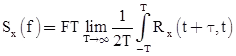• If the two processes X(t) and Y(t) are uncorrected, then Rxy(τ) = E[X(t)] E[Y(t)], where X(t) and Y(t) are jointly stationary processes.
Offer running on EduRev: Apply code STAYHOME200 to get INR 200 off on our premium plan EduRev Infinity!

10 docs|12 tests

,

,

,

,

,

,

,

,

,

,

,

,

,

,

,

,

,

,

,

,

,

;